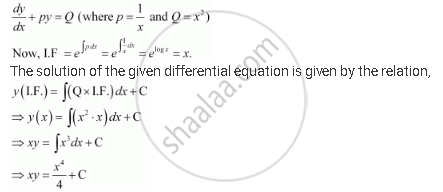Share

# For the Differential Equations Find the General Solution: Dy/Dx + Y/X = X^2 - Mathematics

#### Question

For the differential equations find the general solution:

dy/dx + y/x = x^2

#### Solution

The given differential equation is:This is the required general solution of the given differential equation.

Is there an error in this question or solution?
For the Differential Equations Find the General Solution: Dy/Dx + Y/X = X^2 Concept: Methods of Solving First Order, First Degree Differential Equations - Linear Differential Equations.